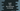# How to find the maximum or largest element in a swift array## How to find the maximum or largest element in a swift array:

In this post, we will learn how to find the largest element in an array in Swift. We can use either array’s max or max(by:) method to find the maximum or largest value in a swift array.

### Definition of max:

max is defined as below:

``func max() -> Element?``

It returns the maximum element in a sequence. If the sequence has no element, it returns nil.

### Examples of max:

Let’s take a look at the below example:

``````let firstArr = [1,2,3,4,5,6]
let secondArr = [1.2,3.4,5.5,6.6,7.7]
let thirdArr = ["a","b","c","d"]
let fourthArr:[Int] = []

print(firstArr.max())
print(secondArr.max())
print(thirdArr.max())
print(fourthArr.max())``````

It will print:

``````Optional(6)
Optional(7.7)
Optional("d")
nil``````

Return values are optional. For the empty array, it returns nil.

### Definition of max(by:):

max(by:) is used for complex objects or if we don’t want the maximum value by natural ordering. This method takes one predicate and based on that predicate it returns the max value. max(by:) is defined as below:

``func max(by areInIncreasingOrder: (Element, Element) throws -> Bool) rethrows -> Element?``

Here, areInIncreasingOrder is the predicate that should returns true if we want the first element before the second element. Else, it should return false.

It returns the maximum value of the array for a non-empty array. Else, it will return nil.

### Example of max(by:) :

Let me show you one example of max(by:) :

``````class Student{
let name: String
let age: Int

init(name: String, age: Int){
self.name = name
self.age = age
}
}
let givenArr = [Student(name: "Alex", age: 10), Student(name: "Bob", age: 11), Student(name: "Chandler", age: 9)]

let maxValue = givenArr.max(by: {(student1, student2)-> Bool in
return student1.age < student2.age
}
)

print(maxValue!.name)``````

Here,

• We have one array of Student objects givenArr.
• The maximum value of the array is calculated by finding out the Student with highest age value.

It will print:

``Bob``